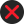19.     Calculation of VAT Payable.           1)      Subject to sub-rule 2,  the tax payable on a taxable sale is calculated by applying the rate of VAT specified in the Act to the sale price of the transaction;           2)      Where the sale price is to be determined under sub-section (2) of Section 11,  the VAT payable shall be calculated by the formula (T x R), where T is the consideration received for the taxable sale and R is the tax fraction.  The consideration minus the VAT calculated by the above formula is the sale price;           3)      The tax payable by a VAT dealer for a tax period shall be calculated by the formula, X-Y where X is a total of the VAT payable in respect of all taxable sales made by the VAT dealer during the tax period, and Y is the total input tax credit the VAT dealer is eligible to claim in the tax period under the Act.           4)      Where any dealer gets himself registered for VAT under sub-section (3) of Section 17, within the time prescribed, the liability for VAT shall be from the effective date of registration,-          *5)   a)  Any VAT dealer opting to pay tax by way of composition under sub-section (9) of Section 4, shall apply for  composition on Form VAT 250 to the prescribed authority.                    b)      Such dealer shall be liable to pay tax at the rate of  twelve and half percent (12.5%) on sixty percent (60%) of the total amount of consideration charged, from the first day of the month in which the application for composition is made. The liability to tax shall continue till the end of the month in which the application for withdrawal of composition is received;                             *( Sub –rule (5) was omitted by the G.O.Ms.nO.1614, Revenue (CT.II), 31st August, 2005 w.e.f 31-8-2005)